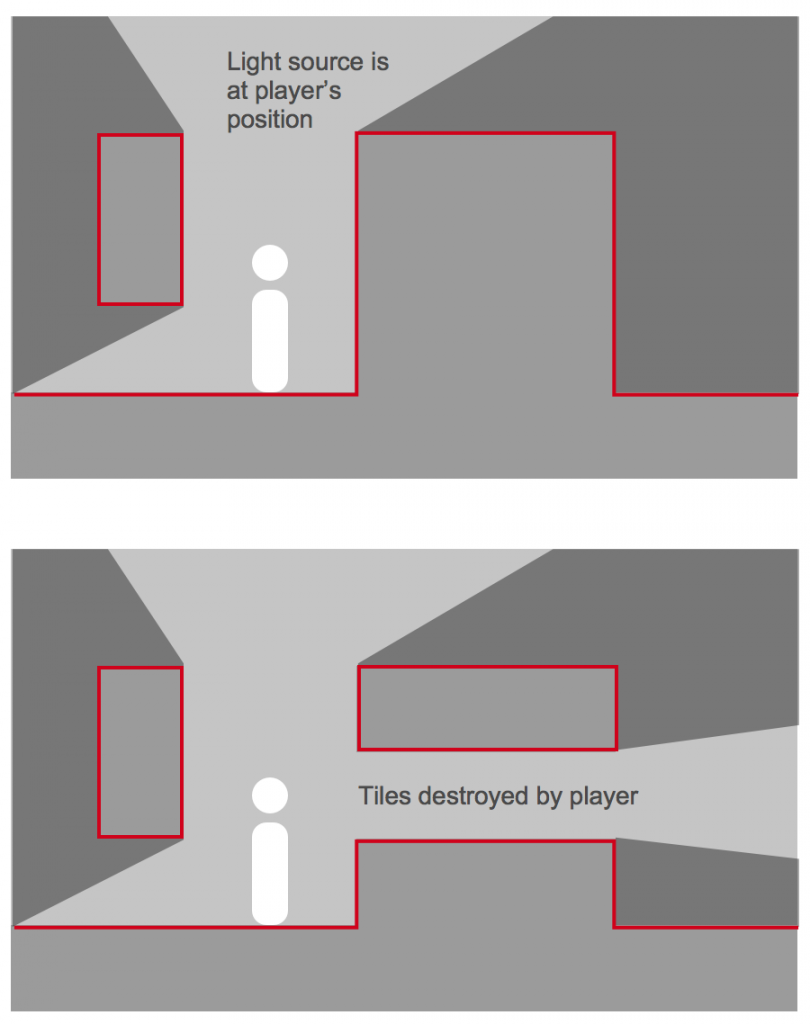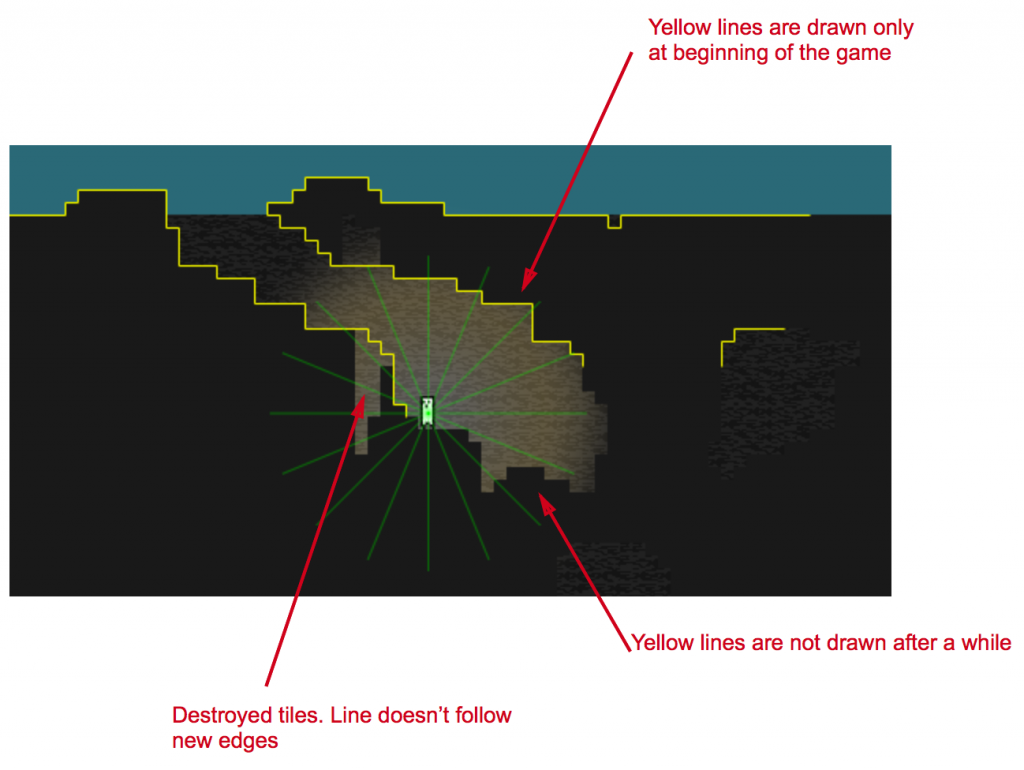# Best approach for raytracing with destroyable tiles and movable light

## Recommended Posts

Hi all,

I've been trying to solve this for hours and I'm looking for broader direction not final code (but that's welcome of course!).

Because I want to add light and shadow (like here: http://gamemechanicexplorer.com/#raycasting-3), I need to draw lines over a tile edge to allow raycasting to find obstacles. In my game I also allow the player to destroy tiles (map.removeTile) so I need to add lines to the adjacent tiles to the one destroyed.

I've been trying to work with TilemapLayer.getTiles(x, y, width, height, collides, interestingFace) in the update method and retrieve an array of tiles that have faces that can be destroyed (interestingFace = true) but the game gets really slow and crashes (even if I limit the area to around the player or to the game size).

What would be the best approach to be able to implement that light and shadow effect within a destroyable world and a movable light source?

Thanks a lot

p.##### Share on other sites

So, I've managed to trace edges of interesting tiles but I have three main issues:

1) lines are drawn only for a while after the game loads, then after some time they are not drawn anymore

2) I player shoots and destroy some tiles the line don't follow the new edges

3) the game is slow

Any help on how improve on those issues?

here the code:

`//called in create();createRays: function() {	this.marker = game.add.graphics();	this.marker.lineStyle(1, 0xFFFF00, 1);	   },//called in update();castRays: function() {//cast rays at intervals in a large circle around the playerfor(var a = 0; a < Math.PI * 2; a += Math.PI/8) {	var ray = new Phaser.Line(this.player.x, this.player.y, 	this.player.x + Math.cos(a) * 100, this.player.y + Math.sin(a) * 100);	//show rays for debug	game.debug.geom(ray, 'rgba(0,255,0, 0.2)');	var intersect = this.getWallIntersection(ray);}},//called in castRays();getWallIntersection: function(ray) {//array with all tiles with interesting facesthis.interestingTiles = this.layerDirt.getRayCastTiles(ray, 1, false, true);for(var i = 0; i < this.interestingTiles.length; i ++) {	//check if tile has interesting faces and draw a line on each	if(this.interestingTiles[i].faceTop) {				this.marker.moveTo(this.interestingTiles[i].worldX, this.interestingTiles[i].worldY);   		this.marker.lineTo(this.interestingTiles[i].worldX+8, this.interestingTiles[i].worldY);	} if(this.interestingTiles[i].faceRight) {			this.marker.moveTo(this.interestingTiles[i].worldX+8, this.interestingTiles[i].worldY);   	this.marker.lineTo(this.interestingTiles[i].worldX+8, this.interestingTiles[i].worldY+8);	}  if(this.interestingTiles[i].faceBottom) {			this.marker.moveTo(this.interestingTiles[i].worldX, this.interestingTiles[i].worldY+8);   	this.marker.lineTo(this.interestingTiles[i].worldX+8, this.interestingTiles[i].worldY+8);	}   if(this.interestingTiles[i].faceLeft) {		       this.marker.moveTo(this.interestingTiles[i].worldX, this.interestingTiles[i].worldY);       this.marker.lineTo(this.interestingTiles[i].worldX, this.interestingTiles[i].worldY+8);		}	}},`##### Share on other sites

Solved all three issues by

a) using Phaser.Line to draw lines (as done in the tutorial I'm following) and game.debug.geom to show lines instead of graphics.line

c) moving the function for drawing line after the function that destroy the tiles, in update

This is the modified code:

`//In create();createRays: function() {				this.marker = game.add.graphics();    this.marker.lineStyle(1, 0xFFFF00, 1);  	},//In update();castRays: function() {    //cast rays at intervals in a large circle around the player    var tiles = [];    //cast ray in a crircle    for(var a = 0; a < Math.PI * 2; a += Math.PI/18) {    	var ray = new Phaser.Line(this.player.x, this.player.y, this.player.x + Math.cos(a) * 100, this.player.y + Math.sin(a) * 100);    			//show rays for debug			game.debug.geom(ray, 'rgba(0,255,0, 0.2)');    }},//In update();traceInterestingTiles: function() {this.interestingTiles = this.layerDirt.getTiles(this.player.x-62, this.player.y-62, 124, 124, false, true);for(var i = 0; i < this.interestingTiles.length; i ++) {		//check if tile has interesting faces and draw a line on each	if(this.interestingTiles[i].faceTop) {			var top = new Phaser.Line(this.interestingTiles[i].worldX, this.interestingTiles[i].worldY,this.interestingTiles[i].worldX+8, this.interestingTiles[i].worldY);   		game.debug.geom(top, 'rgba(0,255,0, 0.3)');	} 	 if(this.interestingTiles[i].faceRight) {				var right = new Phaser.Line(this.interestingTiles[i].worldX+8, this.interestingTiles[i].worldY,this.interestingTiles[i].worldX+8, this.interestingTiles[i].worldY+8);   		game.debug.geom(right, 'rgba(0,255,0, 0.3)');	}	 	if(this.interestingTiles[i].faceBottom) {				var bottom = new Phaser.Line(this.interestingTiles[i].worldX, this.interestingTiles[i].worldY+8, this.interestingTiles[i].worldX+8, this.interestingTiles[i].worldY+8);   		game.debug.geom(bottom, 'rgba(0,255,0, 0.3)');	}	 	if(this.interestingTiles[i].faceLeft) {				var left = new Phaser.Line(this.interestingTiles[i].worldX, this.interestingTiles[i].worldY,this.interestingTiles[i].worldX, this.interestingTiles[i].worldY+8);   		game.debug.geom(left, 'rgba(0,255,0, 0.3)');			}	}},`